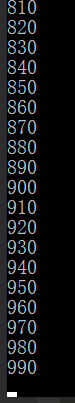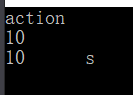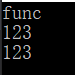# 委托和lambda表达式，Action和Func

## 1、为什么要用委托

public static List<int> GetNum() {
List<int> lst = new List<int>();
//这个算法是最简陋的，但是举这个例子是最合适的
for (int i = 1; i < 1000; i++)
{
if (i%10==0) {
}
}
return lst;
}

public static List<int> GetNum(有一个int类型的参数返回值为Boolean类型的方法) {
List<int> lst = new List<int>();
for (int i = 1; i < 1000; i++)
{
if (有一个int类型的参数返回值为Boolean类型的方法) {
}
}
return lst;
}

## 2、委托如何使用

### 委托其实就是一个能够指向方法的 指针，定义了一个委托就是定义了一个 类型

//访问修饰符  委托关键字  方法的返回值类型  要定义的类型名（参数1，参数2.....）;
public delegate Boolean DelegateFunc(int x);

//类型 变量名 = 实例化一个Object类型的对象(构造函数有无参数)
Object obj = new Object();

//类型       变量名 = 实例化一个DelegateFunc类型的对象(构造函数有无参数)
DelegateFunc func = new DelegateFunc();

//相当于方案1
public static Boolean Condition1(int i)
{
//模拟复杂的操作 相当于return i%10==0;
int x = i % 10;
if (i % 10 == 0)
{
return true;
}
else {
return false;
}
}

//构造函数放入方法的话不需要带()，带()的话是调用
DelegateFunc func = new DelegateFunc(Condition1);

//同样都是类型Object类型可以这样写
//因为String最终是继承自Object，并且String可以默认转换为Object
Object obj = "obj";
//而DelegateFunc也可以通过这种方式赋值，这说明Condition1可以默认转换为委托类型
DelegateFunc func = Condition1;

//调用委托类型的对象和调用普通的方法是一样的
func(10);

public static List<int> GetNum(DelegateFunc func)
{
List<int> lst = new List<int>();
for (int i = 1; i < 1000; i++)
{
//调用传过来的方法，根据调用者传过来的方法拿到想要的数字
if (func(i))
{
}
}
return lst;
}

//声明委托
DelegateFunc func = new DelegateFunc(Condition1);
//调用方法
List<int> lst = GetNum(func);
//也可以直接调用，因为都会默认转换
//List<int> lst = GetNum(Condition1);
//输出
foreach (int item in lst)
{
Console.WriteLine(item);
}//声明一个新的方案2
public static Boolean Condition2(int i) {
//同样模拟复杂的操作 相当于return i%8==0;
int x = i % 8;
if (i % 8 == 0)
{
return true;
}
else {
return false;
}
}

## 3、lambda表达式

### 首先lambda表达式只是方法的一种写法！lambda声明的方法是匿名方法，它和委托并不是绑死的，这是两个东西，但是lambda表达式和委托结合使用是非常常见的！

看一下lambda表达式的语法，也就是函数的另一种写法

//可以这样写
//DelegateFunc func = new DelegateFunc((i) =>
//{
//    return i % 8 == 0;
//});
//也可以这样 不进行new DelegateFunc操作，因为会默认转换
//如果有多句的话，这样写每一句通过分号隔开
DelegateFunc func = (i) => { return i % 8 == 0; };
//如果只有单句 不用写return    默认reuturn   i%8==0 这一句计算出来的值
func = (i) => i % 8 == 0;
//如果只有一个参数   多个参数的话要(i,j,....)这样写
func = i => i % 8 == 0;

//无返回值
public static void HelloWord() => Console.WriteLine("Hello Word!");
//有返回值
public static String GetHelloWord() => "Hello Word!";

//8的倍数
List<int> lst = GetNum((i) => i % 8 == 0);
//10的倍数
lst = GetNum(i => i % 10 == 0);
//20的倍数
lst = GetNum(i => i % 20 == 0);

## 4、Action和Func

Action和Func是微软已经定义好的的两种委托类型，区别是Action是没有返回值的，而Func是需要返回值的

Action使用方法

//无参数
Action action1 = () => Console.WriteLine("action");
//有参数的话调用Action<T>
Action<int> action2 = (i) => Console.WriteLine(i);
//多个参数就在生命的时候<T,T,T>
Action<int,string> action3 = (i, str) => Console.WriteLine(i+"\t"+str);
//调用
action1();
action2(10);
action3(10,"s");Func使用方法

//Func是没有Func类型的，只有Func<T>类型
Func<string> func1 = () => "func";
//如果需要参数 Func<T,T,T>
//最后一个T类型为返回值类型，前面的全都为参数的类型!!!
Func<string, int> func2 = (i) => int.Parse(i);
//如果有多个参数     最后一个T类型为返回值类型，前面的全都为参数的类型!!!
Func<int, string, int> func3 = (i, str) => int.Parse(i + str);

//调用
Console.WriteLine(func1());
Console.WriteLine(func2("123"));
Console.WriteLine(func3(1,"23"));posted @ 2019-08-21 15:44  也难熬  阅读(2937)  评论(5编辑  收藏  举报